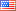# Revision history [back]

### all final(optimal) values of for loop

I have written a program to check which matrices in a given class maximizes the determinant. But my program gives me only one such matrix, I need all those matrices which attains the maximum determinant.

def xyz(n): m=0 A=matrix(QQ,n) for a in myfunction(n): if a.det()>m: m=a.det() A=a print A2 NoneJohn Palmieri7648 ●18 ●66 ●166 http://www.math.washin...

### all final(optimal) values of for loop

I have written a program to check which matrices in a given class maximizes the determinant. But my program gives me only one such matrix, I need all those matrices which attains the maximum determinant.

 def xyz(n):
m=0
A=matrix(QQ,n)
for a in myfunction(n):
if a.det()>m:
m=a.det()
A=a
print  AA